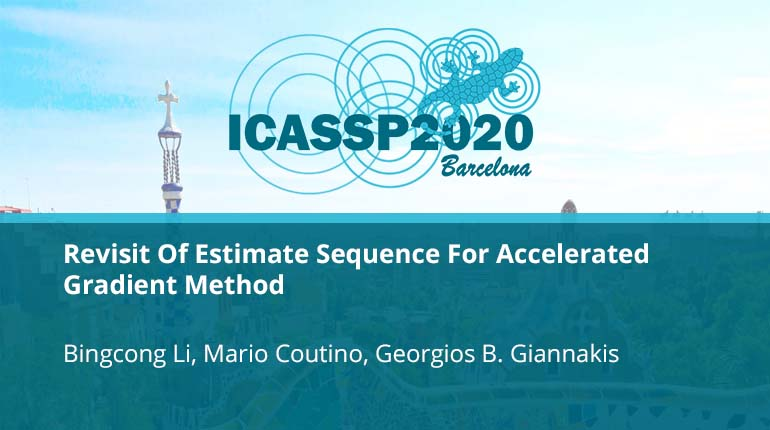# Revisit Of Estimate Sequence For Accelerated Gradient Method

Collection:In this paper, we revisit the problem of minimizing a convex function $f(\mathbf{x})$ with Lipschitz continuous gradient via accelerated gradient methods (AGM). To do so, we consider the so-called estimate sequence (ES), a useful analysis tool for establi
• IEEE MemberUS $11.00 • Society MemberUS$0.00
• IEEE Student MemberUS $11.00 • Non-IEEE MemberUS$15.00
Purchase

## Revisit Of Estimate Sequence For Accelerated Gradient Method

In this paper, we revisit the problem of minimizing a convex function $f(mathbf{x})$ with Lipschitz continuous gradient via accelerated gradient methods (AGM). To do so, we consider the so-called estimate sequence (ES), a useful analysis tool for establi